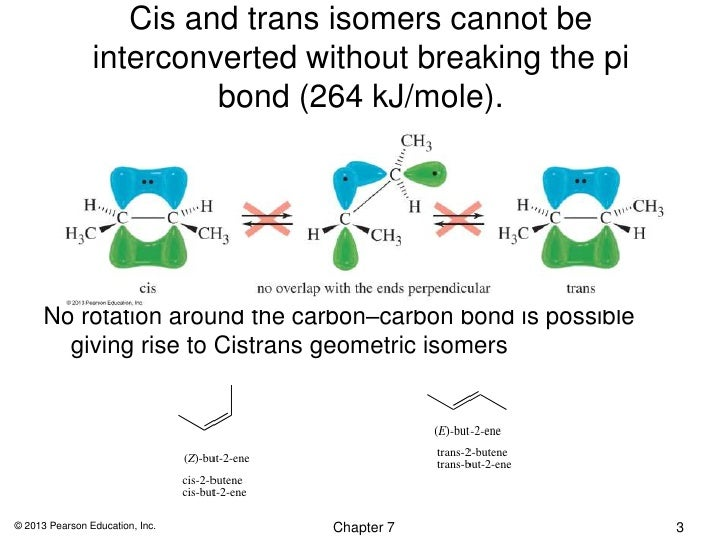# What is "breaking the Pi bond" in alkenes?

##### 1 Answer
Dec 7, 2014

Breaking the π bond in an alkene means twisting one end of the bond relative to the other so that the $p$ orbitals no longer overlap.

A π bond in an alkene forms when the $p$ orbitals of two $s {p}^{2}$ hybridized carbon atoms overlap side-by-side.The most stable arrangement is when the two p orbitals are parallel. This gives the most effective overlap.

As you rotate one $p$ orbital relative to the other, the overlap decreases, and the π bond becomes weaker.When the $p$ orbitals are at right angles to each other, there is no overlap at all. You have broken the π bond.

Breaking the π bond takes energy, so the most stable geometry of the molecule is planar. You can disturb this arrangement only by adding energy.

The result is that there are cis and trans isomers of but-2-ene. A sample of one of these compounds does not become the other. You would have to break the π bond, and there isn't enough energy available to do that.